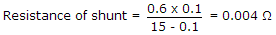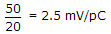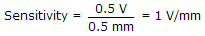# Electronics and Communication Engineering - Measurements and Instrumentation

### Exercise :: Measurements and Instrumentation - Section 1

11.

A moving coil instrument has a resistance of 0.6 Ω and full scale deflection at 0.1 A. To convert it into an ammeter of 0-15 A range, the resistance of shunt should be

 A. 0.6 Ω B. 0.06 Ω C. 0.1 Ω D. 0.004 Ω

Explanation:.

12.

A piezoelectric force transducer has a charge sensitivity of 20 pC/N. It is connected to a charge amplifier and overall gain of transducer and amplifier is 50 mV/N. The gain of amplifier is

 A. 1 mV/pC B. 1.5 mV/pC C. 2.5 mV/pC D. 4 mV/pC

Explanation:.

13.

An LVDT is used to measure displacement. The LVDT feeds a Voltmeter of 0-5 V range through a 250 gain amplifier. For a displacement 0.5 mm the output of LVDT is 2 mV. The sensitivity of instrument is

 A. 0.1 V/mm B. 0.5 V/mm C. 1 V/mm D. 5 V/mm

Explanation:

Final output = 2 x 10-3 x 250 = 0.5 V..

14.

Assertion (A): When a wattmeter is used for low impedance loads, the pressure coil is connected across the load.

Reason (R): A wattmeter measures apparent power.

 A. Both A and R are true and R is correct explanation of A B. Both A and R are true but R is not correct explanation of A C. A is true R is false D. A is false R is true

Explanation:

Wattmeter measures active power.

15.

As per BIS there are 6 accuracy classes of instruments.

 A. True B. False